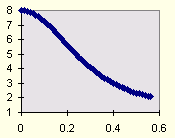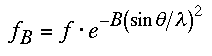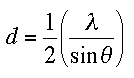# Calculation of atomic scattering factorsThe incoming X-rays are scattered by the electrons of the (protein) atoms. As the wavelength of the X-rays (1.5 to 0.5 A) is of the order of the atom diameter, most of the scattering is in the forward direction. For neutrons of the same wavelength the scattering factor is not angle dependent due to the fact that the atomic nucleus is magnitudes smaller than the electron cloud. It is also obvious that the X-ray scattering power will depend on the number of electrons in the particular atom. The X-ray scattering power of an atom decreases with increasing scattering angle and is higher for heavier atoms. A plot of scattering factor f in units of electrons vs. sin(theta)/lambda shows this behavior. Note that for zero scattering angle the value of f equals the number of electrons.

The normalized scattering curves have been fitted to a 9-parameter equation by Don Cromer and J. Mann . Knowing the 9 coefficients, a(i), b(i) and c, and the wavelength, we can calculate the scattering factor of each atom at any given scattering angle.One must realize though, that the scattering factor contains additional (complex) contributions from anomalous dispersion effects (essentially resonance absorption) which become substantial in the vicinity of the X-ray absorption edge of the scattering atom. These anomalous contributions can be calculated as well and their presence can be exploited in the MAD phasing technique (click here for more on anomalous dispersion). Remember that the i preceeding f" implies that there is a phase shift of +90 deg between f" and the real components of f.In actual cases there will be an additional weakening of the scattering power of the atoms by the so called Temperature-, B- , or Debye-Waller factor. This exponential factor is also angle dependent and effects the high angle reflections substantially (one of the reasons for cryo-cooling crystals is to reduce the attenuation of the high angle reflections due to this B-factor).The B-factor can be related to the mean displacement of a vibrating atom <u> by the Debye-Waller equation

B=8π2<u>2

As u is given in A, the unit for B must be A2. In most protein structures it suffices to assume the isotropic average displacement. At very high resolution with a sufficient variable to parameter ratio, anisotropic refinement of individual temperature factors may be justified. In this case, u is replaced by a 3x3 tensor uij.

# Exercises

The following program lets you calculate the normal scattering factor curves based on Cromer-Mann coefficients.You will see a table of the Cromer-Mann coefficients and plot of the scattering factor in units of electrons vs. sin(theta/lambda) or  resolution in Angstroem (upper labelling of the x-axis frame).  f is calculated once without the use of B and also with the additional attenuation by B. Note how the high angle values for f decrease rapidly with higher B's. B-values for normal regions in protein molecules lie somewhere between 5 (backbone) and 20 (side chains). Note that the resolution is related to wavelength and diffraction angle by the Bragg equation :###Exercise :

Calculate the scattering factor curve for different atoms. See how a high B-factor dramatically decreases the scattering power. What is the value of f for theta = 0 ? What is the effect of high B-factor compared to low occupancy?Click here for the scattering factor calculationBack to X-ray Tutorial Index
This World Wide Web site conceived and maintained by Bernhard Rupp.
Last revised Dezember 27, 2009 01:40